## research papersJOURNAL OFSYNCHROTRONRADIATION
ISSN: 1600-5775
Volume 6| Part 6| November 1999| Pages 1125-1132

## X-ray magnetic circular dichroism with tunable polarization helicity

aLaboratoire de Minéralogie-Cristallographie, Universités Paris 6 et Paris 7, Associé au CNRS, Case 115, 75252 Paris CEDEX 05, France, bInstituto de Física Gleb Wataghin', Universidade Estadual de Campinas, CP 6165, 13083-970 Campinas-SP, Brazil, cLaboratoire Louis Néel, CNRS, BP 166, 38042 Grenoble CEDEX, France, and dLaboratoire de Cristallographie, CNRS, BP 166, F-38042 Grenoble CEDEX, France
*Correspondence e-mail: malgrang@lmcp.jussieu.fr

(Received 11 June 1999; accepted 31 August 1999)

X-ray magnetic circular dichroism spectra have previously been obtained by switching the polarization helicity with a diamond quarter-wave plate (QWP) and keeping the applied magnetic field on the sample as an independent variable [Giles, Malgrange, Goulon, de Bergevin, Vettier, Fontaine, Dartyge & Pizzini (1994). Nucl. Instrum. Methods, A349, 622–625]. These results were obtained by subtracting an undesirable non-magnetic residual spectrum. Experiments reported here show that this residual spectrum mainly originates from a slight lateral displacement of the forward-diffracted beam in the QWP. A quantitative analysis of the phenomenon is in good agreement with predictions from X-ray dynamical theory.

### 1. Introduction

The use of polarized X-rays for the study of microscopic properties of magnetic materials has become popular with the development of two new experimental techniques using synchrotron radiation tuned to the absorption edges of the magnetic atoms. Resonant magnetic X-ray scattering or RMXS (de Bergevin & Brunel, 1972; Gibbs et al., 1988; Vettier, 1994) probes the real part of the index of refraction through scattering whereas X-ray magnetic circular dichroism or XMCD (Schütz et al., 1989; Chen et al., 1990; Dartyge et al., 1992) probes its imaginary part. Both techniques have the advantage of being element- and orbital-specific.

In this paper we will concentrate on the development of an experimental technique improving the capabilities of recording XMCD spectra in the energy-dispersive mode. The X-ray magnetic circular dichroic signal is defined as the difference in absorption for right- and left-handed circularly polarized X-rays by a magnetic sample with the magnetization direction along the photon wavevector. XMCD spectra are generally recorded keeping the polarization helicity constant and reversing the sample magnetization direction by means of an external magnetic field. If one uses a quarter-wave plate (QWP) to transform the linear polarization into circular polarization it is easy to reverse the helicity of the outgoing circular polarization and then to go back to the original definition of the XMCD. Note that in third-generation sources this can be easily performed using helical undulators.

X-ray quarter-wave plates adapted to an energy-dispersive absorption spectrometer have been developed and used for recording XMCD spectra in the 7–11 keV energy range on the DCI synchrotron ring at LURE, Orsay, France (Giles, Malgrange, Goulon, de Bergevin, Vettier, Dartyge et al., 1994). The first XMCD spectra recorded by flipping the polarization helicity of the incoming beam (Giles, Malgrange, Goulon, de Bergevin, Vettier, Fontaine et al., 1994) are presented in Fig. 1. They were measured at the L3 edge of holmium (E0 = 8071 eV) for an HoFe2 Laves phase sample. The spectra obtained for a magnetic field parallel (Fig. 1a) and antiparallel (Fig. 1b) to the photon wavevector are not exactly opposite, as they would be if XMCD was the only contribution to the signal. If we admit that the signal derives from XMCD plus an additional non-magnetic spectrum R, the XMCD can be obtained from the half-difference of spectra (a) and (b) (Fig. 1c), and R from their half-sum (Fig. 1d). The XMCD obtained in this way is identical to that obtained by reversing the direction of the magnetic field. The advantage of this method is that the magnetic field becomes a variable parameter: XMCD spectra can be measured as a function of magnetic field intensity and, for example, element-selective hysteresis cycles can be measured (Pizzini et al., 1997).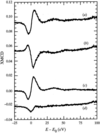Figure 1 XMCD spectra at the Ho L3 absorption edge (E0) of an HoFe2 sample obtained by switching the polarization helicity with a quarter-wave plate. (a) Spectrum obtained with a constant magnetic field parallel to the photon wavevector; (b) spectrum obtained with the magnetic field antiparallel to the photon wavevector; (c) pure XMCD signal without artifacts obtained as the half-difference of spectra (a) and (b); (d) pure residual spectrum R obtained as the half-sum of (a) and (b).

The origin and behaviour of such a residual spectrum has not been understood in detail until now. The series of experiments reported in this paper were performed later in order to analyse the residual spectrum, whose origin is now fully understood.

This paper is organized as follows. In §2we briefly describe the concept of the energy-dispersive spectrometer used to record XMCD with an X-ray quarter-wave plate. §3recalls the principle of the quarter-wave plate. In §4we present experiments which show that two effects are combined to give the residual signal; one is due to the imperfections present in the crystal and the other results from a displacement of the beam analysed using dynamical theory of X-ray diffraction in §5.

### 2. XMCD on an energy-dispersive spectrometer

Measurements were carried out on the energy-dispersive absorption beamline at DCI-LURE (D11).

The principle of the energy-dispersive spectrometer has already been described in detail elsewhere (Tolentino et al., 1988) and will be briefly recalled here. The energy-dispersive set-up uses a curved crystal optics to focus a polychromatic beam onto the sample position coupled to a one-dimensional detector (linear array of 1024 diodes, each 25 µm) allowing the full spectra to be recorded at once. The polychromatic beam has an energy–angle correlation that is transformed into an energy–position correlation on the detector. This allows the full spectra to be obtained without any mechanical movement, i.e. with great stability.

Circularly polarized X-rays can be obtained either from the source (bending magnet or exotic insertion devices) or by transforming the linear polarization state of the incoming beam by a quarter-wave plate. X-ray quarter-wave plates are based on the use of crystal plates near a Bragg diffraction condition (Giles et al., 1993). When such a device is used in the energy-dispersive spectrometer a non-dispersive configuration between the crystal phase plate and the curved crystal polychromator has to be fulfilled in order to simultaneously transform the entire energy bandwidth from linear to circular polarization. This is performed taking into account the fact that the diffraction plane of the quarter-wave plate is set at an angle ψ = 45° from the horizontal plane to allow equal amplitudes of σ and π polarizations in the incoming beam. It has been demonstrated that a careful change of the diffraction plane around the 45° value is essential to fulfill the non-dispersive condition which is given by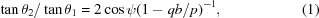where θ1 and θ2 are the Bragg angles on the curved polychromator and the phase plate, respectively, for the mean energy, b is the asymmetry factor related to the angle of asymmetry α of the reflecting planes in the curved polychromator given by b = sin (θα)/sin (θ + α), p is the distance between the source and the crystal and q is the distance between the crystal and the focus point.

Let us call d1 and d2 the lattice plane distances relative to the reflections on the curved Si crystal and the QWP. This condition allows d1/d2 and the angle ψ between the two planes of diffraction to be determined for a given mean energy. A 111 reflection on the curved silicon crystal and a 111 reflection on the diamond crystal are a good combination which give angles ψ ≃ 45° for X-ray energies between 7 and 15 keV. Details of such arrangements are described by Giles, Malgrange, Goulon, de Bergevin, Vettier, Dartyge et al. (1994).

The energy-dispersive set-up has unique advantages when using a quarter-wave plate to record XMCD spectra. First, the QWP is already set for the whole energy range of the XMCD spectra and does not need further angular adjustments. This improves the stability of the set-up and allows measurements of very small dichroic signals. Second, the QWP allows a fast tunability of the polarization helicity of the beam enabling a wide range of experiments in the study of magnetic materials. The principle of the X-ray quarter-wave plate in the transmission mode as used in this work will now be revised.

### 3. Principle of the QWP

The X-ray quarter-wave plates principle relies on the birefringence of perfect crystals close to Bragg diffraction incidence. Several set-ups are possible with different efficiencies and X-ray energy ranges of application. The quarter-wave plate in the transmission mode (i.e. selecting the forward-diffracted beam) used in this work was first proposed by Dmitrienko & Belyakov (1980) and experimentally evidenced using a very well collimated beam by Hirano et al. (1991). The phase shift induced by the phase plate varies with the angle of incidence of the beam on the plate and can be set equal to π/2 or −π/2 by a simple rotation of the phase plate. Starting with incoming linear polarization one can then obtain circular polarization with tunable helicities.

X-ray dynamical theory (Authier, 1961, 1986) shows that there is a difference between σ and π polarization indices of refraction, denoted by nσ and nπ, respectively (Malgrange, 1996). This difference is maximum inside the domain of reflection but it decreases rather slowly outside this domain where the phase shift induced by a plate is given to a very good approximation by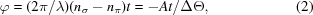with A =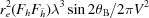.

ΔΘ, called the offset, is the difference between the angle of incidence and the incidence at the middle of the rocking curve. It is often written as ΔΘ = ΔθΔθ0, where Δθ is the departure from exact Bragg incidence and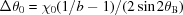, t is the beam path far from Bragg diffraction, b is the asymmetry factor defined in §2, re is the classical electron radius and Fh is the structure factor.

From equation (2)the hyperbolic dependence of the phase shift with the angular offset can be observed. The factor A multiplied by the beam path of X-rays through the crystal determines the angular position that fulfills a 90° phase shift between the σ and π components of the beam. In order to change the sign of the phase shift, and consequently the helicity of the circular polarization, a simple rotation of the crystal from positive to negative values of the offset is necessary. The birefringence is the same for the diffracted and the forward-diffracted beam. It is then very convenient to use, as shown in this work, the forward-diffracted beam which does not change the direction of the beam.

It is also convenient to have an offset value, corresponding to the 90° phase shift, well outside the domain of reflection. Within the reflection domain differences in the transmission profiles for the σ and π components decrease the ellipticity of the transmitted beam. Also, the rate of change of the phase shift φ is quite large increasing the sensitivity of the quarter-wave plate to the divergence of the incident beam. Furthermore, far from the reflection domain only one wavefield is excited and thus either the Laue or Bragg geometry can be used.

The value of the offset that corresponds to a phase shift of 90° can be increased by increasing either the A value or the value of the beam path t [equation (2)]. A is defined by the X-ray energy and the reflection on the QWP crystal. The beam path can be increased keeping a compromise with the transmission through the QWP. This is better achieved using crystals with a low absorption coefficient and this is the reason for using diamond rather than silicon crystals.

For a crystal of given thickness, the beam path t inside the crystal depends on the geometry of the reflection. Here the diamond QWP is a (111) plate, 0.72 mm thick, and a 11¯1 reflection is used in Laue asymmetric geometry. The angle α between the normal to the crystal surface and the incident beam is 19.47° and we chose to use the smaller beam path which corresponds to a beam path almost normal to the crystal surface since the Bragg angle for the 111 reflection on diamond at the Ho L3 edge (λ = 1.536 Å) is equal to 21.9°. Under these conditions the value of the offset satisfying equation (2)for a phase shift equal to π/2 is −43 arcsec. Owing to the divergence of the incident beam, the offset giving the maximum value of the circular polarization rate is larger. For a Gaussian incident beam of FWHM 75 arcsec, the theoretical value is −74 arcsec with a flat maximum. The spectra shown in Figs. 1(a) and 1(b) were taken for non-exactly symmetric offsets of the order of 60–70 arcsec.

### 4. Measurement of XMCD flipping the polarization helicity

#### 4.1. Experimental procedure

The usual procedure of recording an XMCD signal flipping the polarization helicity is to identify the positive and negative offset values (ΔΘ1 and ΔΘ2) of the QWP that produce the highest XMCD signals. These values correspond to the maximum of right- and left-handed circular polarizations. Then the experimental spectrum is recorded as the difference of the absorption spectra obtained with the QWP set at these two offset values keeping the external magnetic field constant.

Previous typical results shown in Fig. 1[curves (a) and (b)] show that the recorded spectrum has an additional signal to the XMCD signal. A clear understanding of the origin of the residual spectrum can be obtained from a detailed analysis of the recording procedure. Let us denote the absorption coefficient and thickness of the QWP and the sample by μq, tq and μs, ts, respectively, and let I0, Iq and Is be the intensities at the exit of the curved polychromator, after the QWP and after the sample, respectively (Fig. 2). Then the transmitted intensities Iq and Is can be expressed by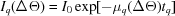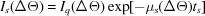where μq, μs, Iq and Is have been explicitly written as functions of ΔΘ to evidence their dependence on the angular offset of the QWP. The dependence of μs on ΔΘ is related to its variation with the sign and amplitude of the circular polarization rate produced by the QWP at offset ΔΘ. The dependence of μq on ΔΘ will be discussed in §4.2.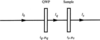Figure 2 Schematic drawing defining the notation used in the text. Iq is the intensity after the QWP, tq and μq are the thickness and absorption coefficient of the QWP. Is is the intensity after the sample and ts and μs are the thickness and absorption coefficient of the sample, respectively.

For the spectrum recorded without the sample, i.e. after the quarter-wave plate only, then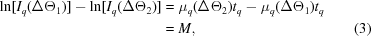and, for the signal after the QWP and the sample,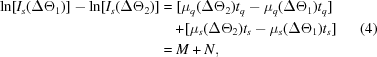where N = μs(ΔΘ2)tsμs(ΔΘ1)ts.

Equation (4)shows that the XMCD spectrum is the sum of two signals: N due to the magnetic sample and proportional to the dichroic signal, and M from the difference in the absorption coefficient of the QWP for two different offset values.

In order to isolate the artifacts in the spectrum solely due to the diamond phase retarder, two series of absorption spectra were recorded, one with a non-magnetic sample containing holmium, HoCo2, the other without any sample. The XMCD-like' spectra were recorded flipping the QWP between a constant large negative offset ΔΘref = −300 arcsec where the artifacts become negligible and several values of the offset ΔΘ including ΔΘ = 0 where X-ray dynamical effects are maximum. These spectra are referred to later as SpJ(ΔΘ),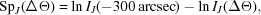where J = Q without the sample [equation (3)] and J = S [equation (4)] with the non-magnetic HoCo2 sample.

Fig. 3shows different SpQ(ΔΘ) recorded with the QWP only. A clear variation of the transmitted intensity through the QWP as a function of the angular offset ΔΘ is evidenced. This signal corresponds to the term M in equation (3)and its origin will be discussed in §4.2.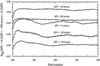Figure 3 Spectra SpQ(ΔΘ) recorded without the sample by flipping the QWP between a constant large negative offset of −300 arcsec and several ΔΘ values (−60, −20, 0, 40 and 80 arcsec).

Subtracting this signal from the signal recorded with a sample should result in an XMCD signal for a magnetic sample or no signal for a non-magnetic sample. The presence of a signal in the difference spectra recorded with a non-magnetic sample is due to an artifact created by the transmission of the beam through the sample and will be analysed in §4.3.

#### 4.2. Influence of the diamond phase plate

The spectra shown in Fig. 3, obtained with the QWP only, present two main features. First, their mean value varies with ΔΘ and, second, some of them present rather large local variations around this mean value.

The mean value variation originates from the transmission profile of the QWP. The full line in Fig. 4represents the transmission curve calculated for a perfect diamond crystal with a diffraction plane at ψ = 42.4° from the horizontal plane and convoluted by a Gaussian function of FWHM 75 arcsec representing the monochromatic divergence of the beam as found by other independent measurements (Giles, 1995).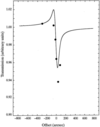Figure 4 Calculated transmission profile (full line) of a perfect diamond crystal, 111 reflection in asymmetric Laue geometry with a diffraction plane inclined by ψ = 42.4° with respect to the horizontal linear polarization direction of the incident beam, convoluted by a Gaussian profile of FWHM 75 arcsec. The filled circles represent the transmitted mean value of the QWP for different offsets ΔΘ by reference to the transmission for ΔΘ = −300 arcsec.

The mean value of the different SpQ(ΔΘ) is plotted in Fig. 4by reference to the transmitted intensity at ΔΘref = −300 arcsec. The agreement is quite good, in view of the fact that the diamond QWP is a good but non-perfect crystal.

In the energy-dispersive set-up the QWP, positioned typically 50 cm from the focus point, is crossed by a divergent X-ray beam. X-rays with different energies traverse different regions of the QWP crystal. In the case of a non-ideally perfect crystal these different regions may have slight misorientations inducing local changes of the offset value, which can create variations in the transmitted intensity. This explains the oscillations particularly enhanced in the spectra for ΔΘ = 0 arcsec and ΔΘ = −20 arcsec (Fig. 3).

To check that the observed variations of the signal are due to crystal defects, the crystal was translated horizontally (towards lower energies) to change the spectral bandwidth illuminating a given part of the crystal. As expected, the main features of the spectrum were also translated towards lower energies.

Thickness inhomogeneities of the QWP may also provoke different transmitted intensities in different regions of the spectrum.

#### 4.3. Evidence of a lateral translation of the beam

In §5we will demonstrate that the mean position of the X-ray beam on the exit surface of the crystal varies with the offset. The incident beam arrives on the QWP at point O (Fig. 8) and would exit at A if the propagation was straight. In fact, the beam exits at A′ whose position depends on ΔΘ. The forward-diffracted beam leaves the crystal parallel to the incident beam but shifted by a length noted x(ΔΘ).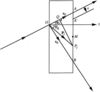Figure 8 Geometrical construction in direct space of the propagation direction of the Poynting vector Pj in the asymmetric Laue case. OAB is the Borrmann fan. A′ is the mean position of a beam giving rise to a forward-diffracted beam whose distance to the incident beam is x.

In this section we will analyse the effects of such a displacement on the difference of two absorption spectra measured for two different offsets. Let us call Δx the lateral shift of the beam when going from one offset, ΔΘ1, to the other, ΔΘ2. It gives the same displacement on the linear detector which is set perpendicular to the beam. A variation of position on the detector is equivalent to a shift in energy. The difference of the two spectra therefore gives rise to a derivative signal. Let us call μsts the spectrum measured for the ΔΘ1 offset and μsts for ΔΘ2. Then,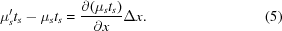∂(μsts)/∂x represents the derivative of the absorption signal with respect to the coordinate x along the linear detector. This is proportional to the derivative with respect to the energy E and is maximum at the absorption edge.

A lateral displacement Δx therefore produces a signal which is proportional to the lateral displacement itself and to the derivative of the absorption spectrum. This will appear as a pseudo-dichroic signal.

In order to evidence this effect we have chosen a non-magnetic sample HoCo2, at room temperature, for which XMCD does not contribute to the difference signal.

From each spectrum SpS(ΔΘ) recorded for the HoCo2 sample we subtracted the spectrum SpQ(ΔΘ) obtained with the QWP only (Figs. 5a and 5b). The result (Fig. 5c) was then compared with a signal proportional to the derivative of the absorption signal (dotted line in Fig. 6a).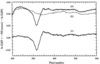Figure 5 (a) Spectrum SpS(ΔΘ = 0) recorded with a non-magnetic sample containing Ho (HoCo2) by flipping the QWP between a constant and large negative offset ΔΘref = −300 arcsec and ΔΘ = 0 arcsec. (b) Spectrum SpQ(ΔΘ = 0) recorded without the sample. (c) Signal obtained as the difference between spectra (a) and (b).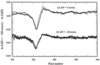Figure 6 (a) Full line: SpS(ΔΘ = 0) − SpQ(ΔΘ = 0) shown also in Fig. 5(c). Dotted line: derivative of the absorption spectrum of the same HoCo2 sample multiplied by a factor corresponding to a displacement of the beam of 17.5 µm. (One pixel corresponds to 25 µm.) (b) Same as (a) for ΔΘ = −20 arcsec and the multiplying factor corresponds to a 11 µm displacement.

From the coefficient of proportionality one can deduce the value of the displacement of the beam on the array of diodes [equation (5)]. For the case ΔΘ = 0 the displacement of the beam has been determined to be equal to 17.5 µm.

The same comparison for the case ΔΘ = −20 arcsec gives a displacement of the beam equal to 11 µm (Fig. 6b).

The different values of the displacements thus determined have been plotted as a function of ΔΘ in Fig. 7. The experimental results show a dependence of the displacement on the offset having its maximum at the centre of the reflection domain. It will be shown below that this is in agreement with dynamical theory.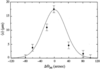Figure 7 Mean lateral displacement (〈x〉) of the beam calculated by X-ray dynamical theory considering a Gaussian angular distribution of the incident beam with FWHM of 75 arcsec (full line). The filled circles correspond to the displacements of the beam deduced from experiments.

### 5. Dynamical theory effect on XMCD spectra

In the case of Bragg diffraction in transmission geometry (Laue case) in a perfect crystal, an incident beam with an offset ΔΘ gives rise to two wavefields 1 and 2 each of which propagates in a direction given by its Poynting vector Pj (j = 1, 2) expressed by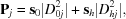where s0 and sh are unit vectors in the incident and reflected directions, respectively, and Dhj and D0j are the amplitudes of the diffracted and the forward-diffracted wave, respectively.

Let us consider an incident beam impinging the crystal at point O (Fig. 8). The incident direction intersects the exit surface of the crystal at point A, and the Poynting vector Pj issued from O intersects the exit surface at point Pj. Let us call M the middle of the basis of the Borrmann fan OAB and Xj the ratio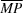j/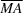. Straightforward geometrical considerations lead to a very simple formula for Xj given below,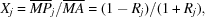where Rj = |γh/γ0|(|Dhj2|/|D0j2|), γ0 and γh are the cosines of the angles ψ0 and ψh between the normal to the crystal surface and the incident and reflected direction, respectively. Let us mention that the asymmetry factor b defined in §2is equal to γ0/γh.

The expression for Rj is very simple when expressed as a function of the usual dimensionless parameter η defined by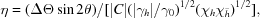with χh = −λ2Fhre/πV, so that,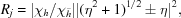where the positive (negative) sign holds for wavefield 1 (wavefield 2).

When Xj is known it is possible to calculate the distance PjQ (Fig. 8) defined as the distance from Pj to OA which is the incident beam direction. Let us call xj the distance PjQ , which can be written as,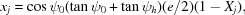where e is the thickness of the QWP.

In order to calculate the correct displacement of the beam in the set-up described here we have to take into account the rather large divergence of the incident beam due to the size of the source. The incident intensity I0 is assumed to have a Gaussian angular distribution I0(ΔΘΔΘm) centred around the mean offset ΔΘm. For each offset the two wavefields propagate giving rise to forward-diffracted waves with an intensity I0j(ΔΘ) = |D0j(ΔΘ)|2 where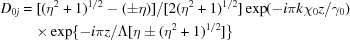where Λ = λ(γ0|γh|)1/2/(χhχ)1/2.

The mean distance 〈x〉 of the beam to a beam propagating without any displacement is given by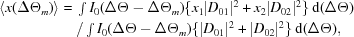where x1, x2, D01 and D02 are functions of ΔΘ.

All the formulae depend on the parameter η which, for a given value of the offset, is different for σ and π polarizations. At the entrance of the crystal the wave is linearly polarized, the polarization vector being inclined by the angle ψ ≃ 45° with respect to the plane of diffraction. This means that the intensity is half σ and half π and this has been taken into account.

The theoretical curves for 〈x(ΔΘm)〉 have been calculated for different values of the FWHM of the Gaussian angular distribution and fitted to the experimental points shown in Fig. 7, as explained in the previous section. The best fit (shown with a full curve in Fig. 7) is obtained for a Gaussian angular distribution whose FWHM is equal to 75 arcsec, in good agreement with the divergence deduced by alternative methods in previous experiments.

The good agreement between this calculation and the experimental points in Fig. 7demonstrates that the residual signal in the XMCD spectra, proportional to the derivative of the absorption spectra, is indeed originated by a lateral displacement of the beam when it crosses the QWP, which varies with ΔΘ.

### 6. Conclusions

The residual signal superimposed on the XMCD spectra when the polarization helicity is switched from right to left by means of a QWP has been systematically studied.

This is interpreted as originating from three different effects. First, the expected variation of the transmission by a perfect crystal as a function of the offset induces a non-zero signal constant along the spectrum. Second, the defects present in the diamond plate may generate a variation of transmission along the plate and therefore a non-constant signal along the spectrum. The third effect originates from the lateral displacement of the beam when propagating in the QWP and gives rise to a signal which is proportional to the derivative of the absorption of the sample. This displacement depends on the offset and can be well understood qualitatively by considering the X-ray propagation inside the Borrmann fan in a perfect crystal. In the symmetric case, for example, at exact Bragg incidence both wavefields propagate along the reflecting planes. For a non-zero offset the two wavefields propagate symmetrically with respect to the reflecting planes but with different intensities. For a large offset only one wavefield carries all the intensity and this wavefield propagates almost along the incident direction. Consequently, for a large offset there is no lateral displacement and, if the incident beam was a plane wave, the normal operating conditions (large offsets) would not give rise to any noticeable displacement of the beam. A displacement was clearly evidenced at the offset of 40 arcsec for example (about eight times the Darwin width) due to the quite large size of the DCI source which generates a Gaussian angular distribution with a FWHM of the order of 75 arcsec.

With third-generation sources like the ESRF where recent experiments performed on beamline ID24 have shown that the FWHM of the incident angular distribution is of the order of 4.5 arcsec (Pizzini et al., 1998), the displacement of the beam at large offsets almost vanishes. However, the residual signal shown in Pizzini et al. (1998) still contains a small modulation around the absorption edge which cannot be described by a derivative signal. This modulation could be due to the defects present in the available diamond plates which could give rise to a small misorientation of the emerging beam, impossible to model. Such an effect would be negligible in the experiments reported here but can be a small contribution in the residual signal shown in Fig. 1.

In summary, accurate XMCD measurements with tunable polarization helicity using a non-perfect quarter-wave plate require the subtraction of the residual signal.

### Acknowledgements

One of us (CG) acknowledges financial support from FAPESP (contract number 96/05586-6), CNPq and PRONEX.

### ReferencesJOURNAL OFSYNCHROTRONRADIATION